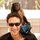Thoughts on Marketing Mix Modeling?

We've recently been exploring Marketing Mix Modeling (MMM) in order to help gauge where some of our unattributed traffic is coming from. Essentially, building a simple linear regression in Python with spend by channel as the independent variables, and total website traffic as the dependent variable.

Here is the current model script.

```# Regress unattributed organic to paid leads to see what fraction of organic
# would be lost if we cut paid spend.

# Factors out bad emails and makes a decent effort to categorize leads by
# referrer and url of first touch.

import sys, scipy, csv
import pandas as pd
import statsmodels.api as sm

script_name = 'regression_mystery_organic_from_paid'
filename = '20161130_us_traffic_reorganized_channels'

df = pd.read_csv('data/' + filename + '.csv', index_col=0)
df.index = pd.to_datetime(df.index)
return df

def ols_summary(df, x_cols, y_col, *m):
if m:
df = df.loc[m]
X = df[x_cols]
y = df[y_col]
est = sm.OLS(y, X).fit()
print_and_save_summary(est)

def print_and_save_summary(est):
summary = est.summary()
output_filename = 'output/' + script_name + '__' + filename
print "\n\nSaving summary to " + output_filename
f = open(output_filename, 'w')
f.write(summary.as_text())
f.close

print summary

if __name__ == "__main__":

Has anyone had any success using the method?

I have been also toying with s-curves, as explained by this blog post: https://www.themarketingtechnologist.co/optimize-media-spends-using-s-response-curves/

I am still very open to ways to optimize this kind of modeling. Any ideas?

Thanks!

4replies Oldest first
• Oldest first